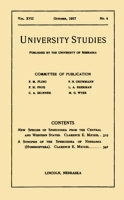## University Studies of the University of Nebraska## Papers from the University Studies series (The University of Nebraska)

1-1905

#### Citation

UNIVERS[TY STUDIES, Vol. V, No.1, January 1905.

#### Abstract

When light polarized at an angle of forty-five degrees to the lines of force is passed through certain dielectrics, between two parallel electrodes, they become double-refracting. The difference of phase, delta, between the two components of light at right angles and parallel to the lines of force, as represented by Kerr and verified by later investigators, is

δ = ± BP2l/a2

where B is the electro-optic constant, depending on, the dielectric, I the length, and a the distance between the plate' electrodes in centimeters, and P the difference of potential between the electrodes in C.G.S. units.

From data obtained in this investigation, there seems to be a decided variation from the above law for low potentials. As the potential was decreased from about 200 volts per millimeter of distance between the electrodes, the decrease of δ was in a smaller ratio than the decrease of P2.

COinS